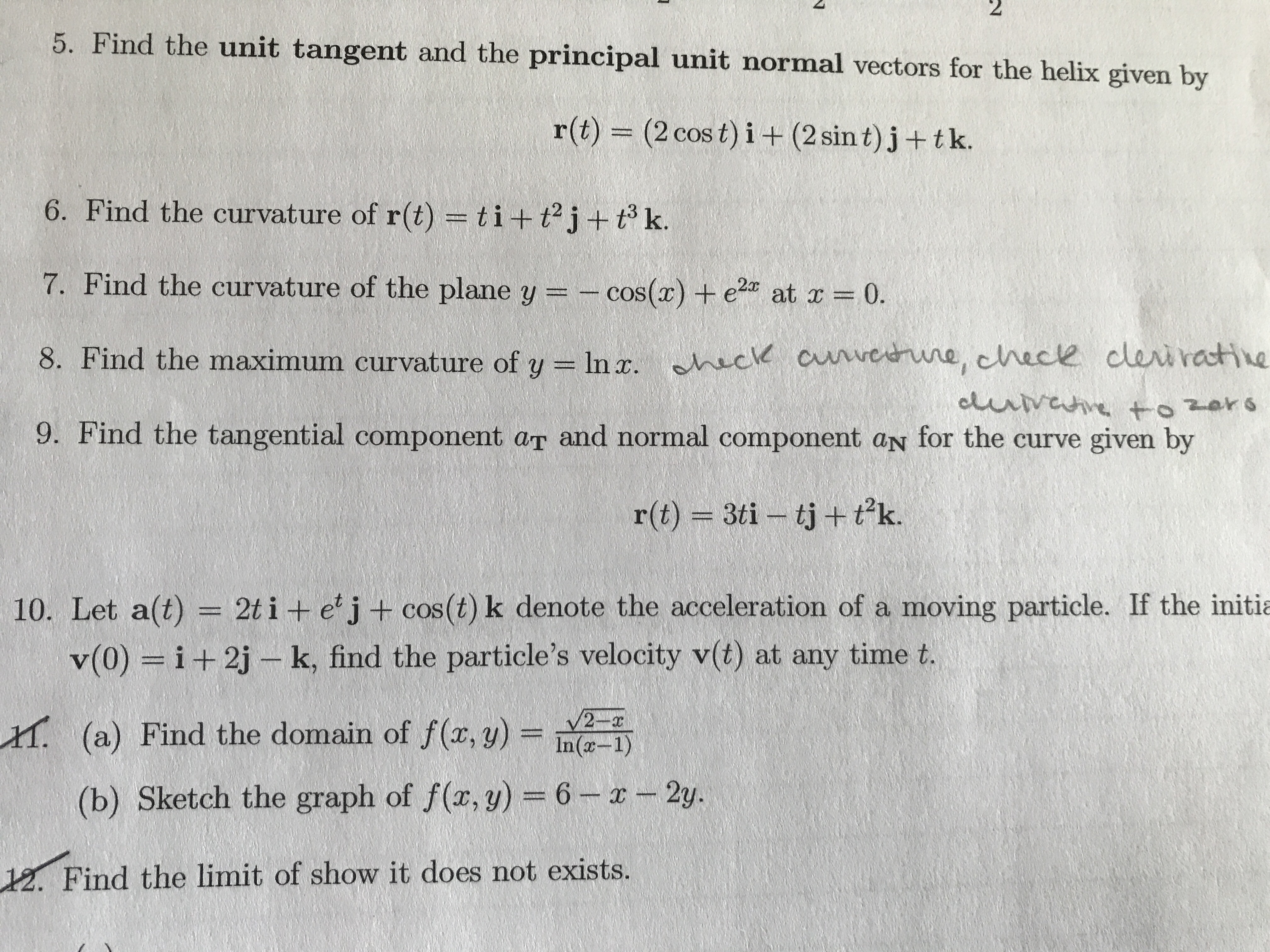# $2 5. Find the unit tangent and the principal unit normal vectors for the helix given by r(t) = (2 cos t) i + (2 sin t) j+ tk. 6. Find the curvature of r(t)= ti+t2j + t k. 7. Find the curvature of the plane y = cos() +e 2x at r 0. 8. Find the maximum curvature of y In . ck aveerune, chece cleiractie eluvahe +ozors 9. Find the tangential component ar and normal component an for the curve given by r(t) 3ti tj+ tk. 10. Let a(t) = 2t i + e' j + cos(t) k denote the acceleration of a moving particle. If the initia v(0) = i+ 2j-k, find the particle's velocity v(t) at any time t. V2 x (a) Find the domain of f(x, y)=In(-1) (b) Sketch the graph of f(x, y) = 6 -2y 12. Find the limit of show it does not exists. Question Help with #5help_outlineImage Transcriptionclose$2 5. Find the unit tangent and the principal unit normal vectors for the helix given by r(t) = (2 cos t) i + (2 sin t) j+ tk. 6. Find the curvature of r(t)= ti+t2j + t k. 7. Find the curvature of the plane y = cos() +e 2x at r 0. 8. Find the maximum curvature of y In . ck aveerune, chece cleiractie eluvahe +ozors 9. Find the tangential component ar and normal component an for the curve given by r(t) 3ti tj+ tk. 10. Let a(t) = 2t i + e' j + cos(t) k denote the acceleration of a moving particle. If the initia v(0) = i+ 2j-k, find the particle's velocity v(t) at any time t. V2 x (a) Find the domain of f(x, y)=In(-1) (b) Sketch the graph of f(x, y) = 6 -2y 12. Find the limit of show it does not exists. fullscreen

### Want to see this answer and more?

Experts are waiting 24/7 to provide step-by-step solutions in as fast as 30 minutes!*

*Response times vary by subject and question complexity. Median response time is 34 minutes and may be longer for new subjects.
Tagged in
MathCalculus

### Derivative